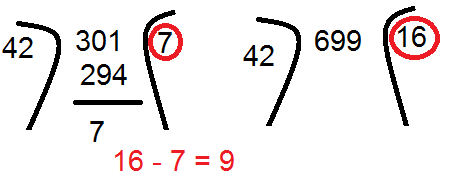# Factors

·       A Prime Number has exactly two factor.
[2,3,5,7,11,13,17,19,23,29,31,37,41,43,47,53,59,61,67,71,73,79,83,89,97] -----Total 25                                          between 1 to 100
·         A Composite Number has more than two factors.
[4,6,8,9,10………………………….] ------Total 74 between 1 to 100

Let us take a number, 15.
Factors of 15 are 1,3,5,15
·         Total no of factors = 4
·         Total no of Prime Factors = 2
·         Total no of different Prime factors = 2

Now, we will learn how to find out no of factors of a no.
N = Ap × Bq  ………………..(1)
No of Factors = (p+1)(q+1)
Any real number can be written in the form of Equation (1)
Example:
15 = 51×31
Hence of factors of number 15 are (1+1)×(1+1)= 4
No of prime factors = 2 (5 & 3)
60 = 2×2×3×5
= 22×31×51
Hence of factors of 60 are (2+1)(1+1)(1+1) =12
No of prime factors = 4 (2,2,3,5)
No of different prime factors = 3(2,2,3,5)

Points to Remember:
·         To find out number of Odd factors, consider only Odd prime no
Example:
60 = 2×2×3×5
= 22×31×51
No of Odd factors = (1+1)(1+1) = 4
Here we have considered only odd prime numbers i.e. 3 & 5.
No of Even Factors = Total factors – Odd Factors

Power of Prime no.
·         Power of P in n! = n/p1 +n/p2 +n/p3 + ……..
·         Only integer part of each term is considered.
·         Terms are considered up to the place results are greater than 1 .
Example:
Power of 2 in 4!
= 4/2 + 4/22 +4/23
= 2 + 1 + 0 (since the value of third term is less than 1)
= 3
Example:
Power of 3 in 80!
= 80/31 +80/32 +80/33 + 80/34
= 26.667+8.889+2.9629+0.9876
= 26+8+2+0 (Only integer parts are considered)
=36

Problem:
How many numbers between 300 and 700 , which are exactly divisible by 2,3 & 7 together.
Solution:
Between 300 to 700 i.e from 301 to 699
Step 1:
Take LCM of 2,3 & 7 that comes out to be 42
Step 2:
Divide First and second number by 42

Step 3:
Ignore the remainder and subtract the result of both division. This will give you the no of numbers.

Hence the no of such numbers between 300 & 700 are 9